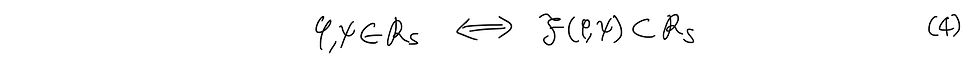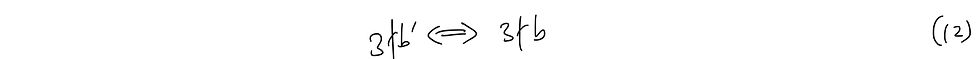top of page
All Posts: Blog2

# [Math Evenings] Banach-Tarski Revisited 4: Applying 2 and 3 to a Sphere I

Until post 3, it is unclear how thm 2 can be applied into a sphere; here's how.

Consider a sphere S^3 defined asThen, also consider set of all rotational motions on the sphere S^2 defined as R_s. The rotational matrices would have a determinant of 1 by definition, and they would form a group. In other words,Each of the rotational movements can be defined as the following as well. (eq 3.1, 2, 3, 4) Phi represents arccos(1/3) anticlockwise rotation by the x-axis. Small pitchfork represents the same rotation, but by the z-axis. The rotations by the y-axis can be obtained by a combination of the phis and pitchforks, as R_s is a group.Now with this preconditions, we will prove theorem 3.

Theorem 3.

Prop. F(phi, pitchfork) is a free group.

To prove this claim, we must prove three lemmas first.

Lemma 3.1. F(phi, pitchfork) is a subset of R_S.

Pf. The proof for this is quite simple, asQ.E.D

Lemma 3.2.

Let there exist an element of F(phi, pitchfork), rho such that it has a length of n. Then, there exists some integers a, b, c such thatPf. We will apply mathematical induction.

Base case: Trivial at n=0

Let us assume that lemma 3.2 holds true at n=k.

Then there would also exist some rho' that has length k-1, asAlso, because we assumed that lemma 3.2 is true at n=k,would also hold true. This means that as we discussed in theorem 2.Note then, by definition, rho is one of eq 9.1, 2, 3, or 4.We assumed that the lemma holds true for rho', and hence every a, b, c will be an integer.

Q.E.D

Lemma 3.3. Especially, b is not a factor of 3.

Pf. We apply the same method as our proof in lemma 3.2, which allows us to express rho asAmong these, we pick an example as in eq 11.Note thatand hence, applying the same method for each of the 12 cases in (10), b cannot be a factor of 3.

Q.E.D

Now, armed with all of these, we will prove theorem 3!

Pf. To prove that F(phi, pitchfork) is a free group, it is sufficient to prove that there does not exist a non-trivial unit element within F(phi, pitchfork).

We will use proof by contradiction, by assuming that there exists some non-trivial unit element rho. Then, it must be true that rho applied to (1,0,0) equals (1,0,0) itself.

Because of lemma 3.2,This means thatbecause of lemma 3.1. But, from lemma 3.3, b cannot be a divisor of 3, and this is a contradiction. Hence, F(phi, pitchfork) is a free group on R_s.

Q.E.D.

This was a really long and tedious proof, but it is truly magnificent to see how this can blossom into a proof for the Banach-Tarski Paradox.

22 views

See All
bottom of page